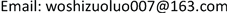1. 引言

2. 物理基础2.1. 相干合成的物理基础

U ( x , y ) = exp ( j k z ) j λ z exp [ j k 2 z ( x 2 + y 2 ) ] a b   sinc ( a x λ z ) sinc ( b y λ z ) (1)

U 1 ( x , y ) = exp ( j k z ) j λ z exp [ j k 2 z ( x 2 + y 2 ) ] a b   sinc ( a x λ z ) sinc ( b y λ z )                                                                                           ⋮ U n ( x , y ) = exp ( j k z ) j λ z exp [ j k 2 z ( x 2 + y 2 ) ] a b   sinc ( a x λ z ) sinc ( b y λ z ) η ( Δ x n , Δ y n ) (2)

I = | U 1 ( x , y ) + ⋯ + U n ( x , y ) | 2 = | exp ( j k z ) j λ z exp [ j k 2 z ( x 2 + y 2 ) ] a b sinc ( a x λ z ) sinc ( b y λ z ) + ⋯     + exp ( j k z ) j λ z exp [ j k 2 z ( x 2 + y 2 ) ] a b sinc ( a x λ z ) sinc ( b y λ z ) η ( Δ x n , Δ y n ) | 2 = | exp ( j k z ) j λ z exp [ j k 2 z ( x 2 + y 2 ) ] a b sinc ( a x λ z ) sinc ( b y λ z ) ( 1 + ∑ i = 2 n η ( Δ x n , Δ y n ) ) | 2 = a 2 b 2 sinc 2 ( a x λ z ) sinc 2 ( b y λ z ) | ( 1 + ∑ i = 2 n η ( Δ x n , Δ y n ) ) | 2 (3)

2.2. 激光锁模的物理基础

E ( t ) = A ( t ) e i ( ω 0 t + φ 0 ) (5)

A ( t ) = E 0 sin 1 2 ( 2 N + 1 ) ( Ω t + β ) sin 1 2 ( Ω t + β ) (6)

I ( t ) ∝ A 2 ( t ) = E 0 2 sin 2 1 2 ( 2 N + 1 ) ( Ω t + β ) sin 2 1 2 ( Ω t + β ) (7)

I ∝ ( 2 N + 1 ) E 0 2 (8)

I m ∝ E 0 2 lim ( Ω t + β ) → 2 m π sin 2 1 2 ( 2 N + 1 ) ( Ω t + β ) sin 2 1 2 ( Ω t + β ) = ( 2 N + 1 ) 2 E 0 2 (9)

2.3. 相干合成与激光锁模的异同

1) 相干合成在空间上实现了能量的汇聚；

2) 相干合成针对同一频率的光束进行相位锁定；

3) 相干合成通常针对谐振腔外的光束进行锁相；

3. 实现相干合成和激光锁模的技术手段分析3.1. 实现激光光束相干合成的技术手段

1) 被动相干合成通过自组织原理在激光器内部实现多路激光的相位锁定，其实现方式主要有干涉仪方式、非线性自组织方式、倏逝波耦合方式等。往往以复合振荡腔的形式存在。被动式相干合成目前达到的效果不是很好，高能激光系统还比较少见  。

2) 主动相干合成利用一定的控制算法通过相位调制器件在激光器外部实现多路激光的相位锁定，控制方法主要有外差法、自适应锁相法、多抖动法、SPGD算法等  。

3.2. 实现激光锁模的技术手段

1) 主动锁模技术可以分为振幅调制锁模、相位调制锁模以及同步泵浦锁模三种。对于前两种，通常是在腔内置入适当的损耗调制或相位调制元件并控制调制的频率从而实现模式锁定。常用的调制器件是声光和电光损耗调制以及电光相位调制。如果使用一台锁模激光器作为泵浦源，对另一台锁模激光器进行泵浦，通过控制谐振腔内的激光增益，来实现锁模脉冲的输出，这种方法则是同步泵浦锁模方法  。

2) 被动锁模技术。被动锁模技术的种类很多，常用的有自锁模(克尔透镜锁模)、附加脉冲锁模以及可饱和吸收体锁模等。相比于自锁模，附加脉冲锁模技术能够实现自启动，可适用于大多数的固体激光介质  。

4. 技术借鉴的可能性分析

4.1. 将成熟的锁模技术应用到相干合成中的可能性

4.2. 相干合成技术在锁模中应用的可能性

1) 激光相干合成的光束在谐振腔外的自由空间，激光锁模针对的光束是在谐振腔内部。若要将成熟的激光锁模技术应用在光束相干合成上面，需要解决如何不在谐振腔里使用被动锁模的技术问题。

2) 激光相干合成的光束在空间上是分离的，激光锁模针对的光束在空间上是纠缠在一起的。若要将成熟的激光相干合成技术应用在激光锁模上面，则需要解决如何分离不同模式的光束，同时加入相位控制单元的问题。

5. 小结